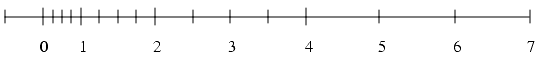## Calculating floating point epsilon/precision in Java

Floating point arithmetic/rounding can be somewhat painful, made worse by the fact that the epsilon (error) in the machine representation of floating point varies depending on the exponent (i.e. how large/small the number being represented is):Normalized numbers when Base = 2, Precision = 3, Exponents = -1 to 2 [Goldberg91]

A very comprehensive paper (from which the above image is taken) is available here. In previous teams we have implemented our own functions to derive an approximation of the epsilon from the number to be represented, however I discovered recently to my surprise that such a function was added to Java in 1.5: Math.ulp!